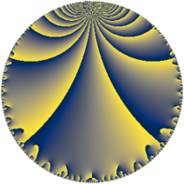Properties

 Label 29.6.eLevel $29$ Weight $6$ Character orbit 29.e Rep. character $\chi_{29}(4,\cdot)$ Character field $\Q(\zeta_{14})$ Dimension $72$ Newform subspaces $1$ Sturm bound $15$ Trace bound $0$

Related objects

Defining parameters

 Level: $$N$$ $$=$$ $$29$$ Weight: $$k$$ $$=$$ $$6$$ Character orbit: $$[\chi]$$ $$=$$ 29.e (of order $$14$$ and degree $$6$$) Character conductor: $$\operatorname{cond}(\chi)$$ $$=$$ $$29$$ Character field: $$\Q(\zeta_{14})$$ Newform subspaces: $$1$$ Sturm bound: $$15$$ Trace bound: $$0$$

Dimensions

The following table gives the dimensions of various subspaces of $$M_{6}(29, [\chi])$$.

Total New Old
Modular forms 84 84 0
Cusp forms 72 72 0
Eisenstein series 12 12 0

Trace form

 $$72 q - 7 q^{2} - 7 q^{3} + 165 q^{4} - 53 q^{5} - 311 q^{6} - 27 q^{7} - 1498 q^{8} + 1567 q^{9} + O(q^{10})$$ $$72 q - 7 q^{2} - 7 q^{3} + 165 q^{4} - 53 q^{5} - 311 q^{6} - 27 q^{7} - 1498 q^{8} + 1567 q^{9} - 7 q^{10} - 2121 q^{11} + 465 q^{13} - 7 q^{14} - 1393 q^{15} - 2811 q^{16} + 13272 q^{18} - 7 q^{19} - 9835 q^{20} + 10493 q^{21} + 8854 q^{22} - 2093 q^{23} + 19949 q^{24} + 1203 q^{25} + 3409 q^{26} - 31255 q^{27} - 25932 q^{28} - 21905 q^{29} - 13118 q^{30} - 2877 q^{31} - 19124 q^{32} + 10087 q^{33} + 60801 q^{34} + 39715 q^{35} + 42694 q^{36} + 16891 q^{37} - 17436 q^{38} - 106659 q^{39} - 56455 q^{40} + 117916 q^{42} + 12327 q^{43} - 142450 q^{44} - 86106 q^{45} + 55433 q^{47} + 301014 q^{48} - 8525 q^{49} + 35882 q^{50} - 56972 q^{51} + 103800 q^{52} + 63572 q^{53} + 88044 q^{54} - 230643 q^{55} - 277767 q^{56} - 69390 q^{57} - 286395 q^{58} + 257244 q^{59} - 218008 q^{60} - 35791 q^{61} - 209605 q^{62} + 25691 q^{63} + 165190 q^{64} + 223392 q^{65} + 509789 q^{66} + 112131 q^{67} + 90580 q^{68} + 216181 q^{69} + 201747 q^{71} - 736925 q^{72} - 281428 q^{73} + 262624 q^{74} + 781767 q^{76} + 294805 q^{77} - 68783 q^{78} - 296583 q^{79} - 356826 q^{80} + 148971 q^{81} + 76830 q^{82} - 52659 q^{83} - 1077531 q^{84} - 351246 q^{85} - 281140 q^{86} - 308849 q^{87} - 154298 q^{88} - 507241 q^{89} - 35378 q^{90} - 18227 q^{91} + 38900 q^{92} + 329531 q^{93} - 581204 q^{94} + 786233 q^{95} + 308366 q^{96} - 640808 q^{97} + 674940 q^{98} + O(q^{100})$$

Decomposition of $$S_{6}^{\mathrm{new}}(29, [\chi])$$ into newform subspaces

Label Dim. $$A$$ Field CM Traces $q$-expansion
$a_{2}$ $a_{3}$ $a_{5}$ $a_{7}$
29.6.e.a $72$ $4.651$ None $$-7$$ $$-7$$ $$-53$$ $$-27$$